Journal of Vibroengineering

Published: 30 September 2018

# The influence of tram tracks on car vehicle speed and noise emission at four-approach intersections located on multilane arteries in cities

Aleksander Sobota1
Renata Żochowska2
Emilian Szczepański3
Paweł Gołda4
1, 2Silesian University of Technology Faculty of Transport, Katowice, Poland
3Warsaw University of Technology Faculty of Transport, Warsaw, Poland
4Air Force Institute of Technology, Warsaw, Poland
Corresponding Author:
Aleksander Sobota
Views 237

#### Abstract

In the article the mathematical models and results of research conducted at the intersection located on multilane artery in big city in Poland have been presented. The research allowed to describe the relationship between the speed of car vehicles and the occurrence of the tram tracks, which may be important in determining the empirical capacity of intersections located on multilane arteries in cities. This dependency may be used when updating the methods of estimation of the capacity of road intersections in Poland , what is the original achievement of the authors. Moreover, the results of research of noise emission in the aspect of speed change of car vehicles and the occurrence of the tram tracks have been presented. In addition, the research problem is of particular importance in the process of selecting the intersection type, which is very important during the planning and organizing traffic at intersections located in cities, where the development of the tram infrastructure is being observed.

## 1. Introduction

Functioning of transport system is determined by the quality of service realized by infrastructure of different transport branches. In case of road transport, the intersections are very important. These objects are usually the bottlenecks in the network. Therefore, the correct selection of the intersection type is really important in the planning and projecting process of infrastructure. The influence on selecting of intersection type is dependent on a lot of factors, that can be grouped into five groups like: factors related with traffic conditions, infrastructure, environment, traffic safety and costs. In addition, in the process of selecting the intersection type, it is necessary to provide services to other users of the transport system, i.e. pedestrians, cyclists or tram passengers.

However, it should be borne in mind that between individual intersection users occurs interactions, which are visible even if one of them is not currently present at the intersection. This situation is especially noticeable in relation to vehicles that pass the intersection through the tram infrastructure it may influence the values of the characteristics describing the factors, on which the choice of the type of road crossing is dependent. That is why, it is important to know the functional relationships describing these interactions. In the paper, the impact of tram tracks on the speed of vehicles, as well as the noise emission level, have been presented.

## 2. Literature review

Designing transport systems requires taking into account many factors and is a multi-criteria issue. Currently, the trend prevails for the design of environmentally friendly solutions that are also effective in terms of time, reliability and quality of service . The functioning of the transport system is associated with its negative impact on the environment. It is mainly manifested by the emission of exhaust gases into the atmosphere and excessive noise.

The problem of the impact of noise on the lives of residents in the city is described by Badyda , Fuks K. , Galilea de Dios Ortuzar . The conducted research concerns mainly the impact of noise on health, as well as the methods of measuring or noise levels depending on the road conditions. There are also many models of noise emission estimation, can be mentioned here: CoRTN , RLS 90 , TRANEX , or used in this work FHWA . These models were often developed several or dozens years ago, but they are updated and often used in practice.

Car vehicle speed as one of the most important measures of traffic condition assessment is the subject of many studies [10-15]. It is a factor affecting both quantitative parameters (e.g. capacity, efficiency) [16, 17] and qualitative ones (e.g. reliability, safety, characteristics of the natural environment), and describing the functioning of point and linear elements of transportation network [18-20]. In the case of an intersection with traffic lights, average speed is an important parameter affecting its capacity and efficiency. Bonneson  developed speed models as a function of the vehicle position in the queue at the signalized intersection. In turn, in other publications [22, 23] the queue discharge speed has been expressed in relation to the time since the start of the displayed green period.

When analyzing the vehicle speed at intersections with traffic lights, it was found that it depends on many geometric and traffic factors of the infrastructure elements (e.g. width of lanes, slope of the inlet, turning radius, generic structure of vehicles, traffic organization) . The quality and condition of the surface may also be included among the factors that significantly affect the speed of traffic. The presence of a tram tracks may therefore significantly worsen the traffic conditions at the intersection.

Average speed of vehicle passing the intersection is one of the most important determinant of saturation flow rate [24, 25]. The speed may decrease by up to 20-30 km/h while passing tram tracks, when the surface is in poor condition. The saturation flow rate is lower by 15 % compared to intersections with rubber-cord surface at tram crossings instead of asphalt .

In the method of estimating the capacity of intersection with traffic lights used in Poland  the impact of passing through the tram tracks is taken into account. In this method, the following formula (Eq. (1)) is used to calculate the saturation flow rate ${S}_{r}$ of a collision-free turn maneuver:

1

where: ${S}_{0}$ – base saturation flow rate [veh/hg], $w$ width of lane [m], $i$ – average slope of the inlet in the distance of 30 m from the stop line [%], ${\delta }_{i}$ – coefficient of slope direction [-], ${\delta }_{k}$ – coefficient of lane location [-], ${\delta }_{t}$ – coefficient of passing through the tram tracks [-], $R$ – turning radius [m], ${u}_{c}$ – share of heavy vehicles [-].

As may be seen, the Eq. (1) takes into account the occurrence of the tram tracks through the coefficient ${\delta }_{t}$, which is equal to 1, when the trajectory of vehicles intersects the tram tracks or to 0, if it does not cross the tram tracks or its technical condition does not significantly reduce the speed of vehicles. This is due to the fact that passing through the tram tracks may cause the change in the speed of vehicles, and thus it may affect the capacity of intersection.

Fig. 1 presents the values of saturation flow rate for collision-free turn maneuver both for occurrence $\left({S}_{r}^{+t}\right)$ and for lack of tram tracks $\left({S}_{r}^{-t}\right)$ in trajectories of car vehicles assuming the same values of the other geometric and traffic characteristics of the intersection (lane width, slope of the inlet, direction of the slope, coefficient of lane location). Such calculations have been made using the formula (Eq. (1)). According to the regulation  it was assumed that the turning radius is between 10 and 40 m.

Fig. 1Saturation flow rate for collision-free turn maneuver as a function of turning radius with different share of heavy vehicles for predetermined base saturation flow rate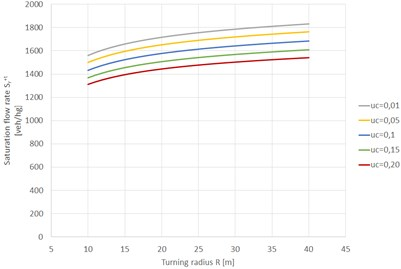a) With tram tracks and for base saturation flow rate ${S}_{0}=$ 1900 veh/hg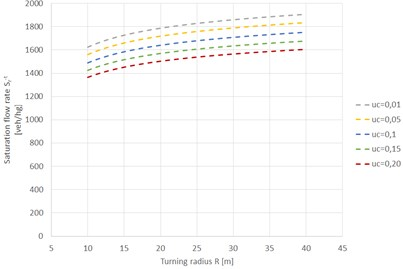b) Without tram tracks and for base saturation flow rate ${S}_{0}=$ 1900 veh/hg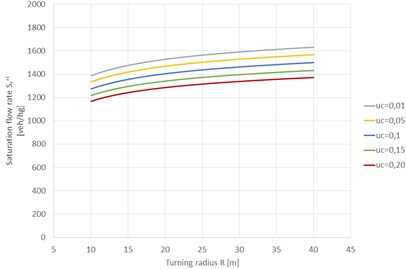c) With tram tracks and for base saturation flow rate ${S}_{0}=$ 1700 veh/hg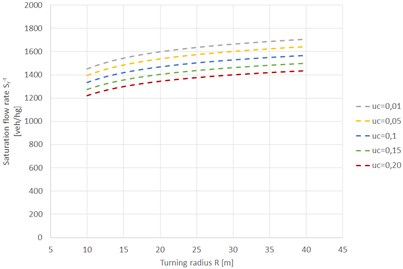d) Without tram tracks and for base saturation flow rate ${S}_{0}=$ 1700 veh/hg

According to the formula (Eq. (1)), the difference $∆{S}_{r}$ between saturation flow rates without and with the tram tracks in trajectories of car vehicles depends only on the turning radius and on the share of heavy vehicles, and it is equal to:

2

Depending on the adopted limits of the turning radius, the difference $∆{S}_{r}$ takes values from about 20 veh/hg to about 80 veh/hg (Fig. 2). The increase in the share of heavy vehicles affects the decrease in saturation flow rates. The highest saturation flow rate occurs with the smallest share of heavy vehicles irrespective of the base saturation flow rate.

It is worth noting that the method of estimation of intersection capacity used in Poland  does not take into account the occurrence of tram tracks when determining the saturation flow rate for straight maneuver, collision left turn maneuver or collision maneuver with pedestrian traffic.

The author of the dissertation  identified 71 factors affecting the capacity of different kinds of intersections, i.e.: intersections with traffic lights, intersections without traffic lights and roundabouts. The factors were then classified into several groups: geometric, traffic, associated with the control method, associated with the organization of traffic, exploitation and weather, local and other. The classification has been made on the basis of the analysis of the capacity estimation methods used in the USA , Germany , Canada  and Poland [1, 27, 33]. However, it should be noted that in these methods factors related to the passing through the tram track by car vehicle are not always taken into account.

Fig. 2Difference between saturation flow rates without and with tram tracks in trajectories of car vehicles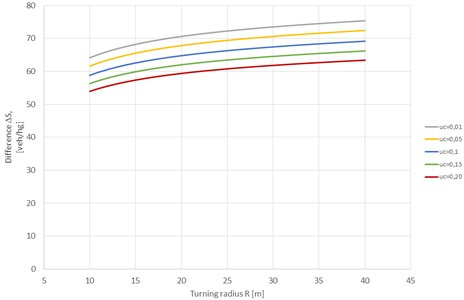## 3. Research method

Prior to performing the studies, a research objects have been chosen, which due to the specificity of the studied phenomenon had to be characterized by the following: state of saturation for individual lanes and inlets, traffic lights, tram tracks, small values of slopes of inlets, the same width of lanes on all inlets.

In order to exclude the influence of the turning radius on vehicle speed, only vehicles traveling in a straight maneuver have been examined. The measurement was carried out in four cross-sections in order to take into account the influence of the distance between the tram tracks and the stop line on the speed of car vehicles.

Fig. 3 shows photographs of example of the intersection with the organization of traffic on the inlets and the numbers and names of individual inlets. The measurement points and cross-sections have been also presented.

Fig. 3Location of measurement points and cross-sections [own source on the basis of 34]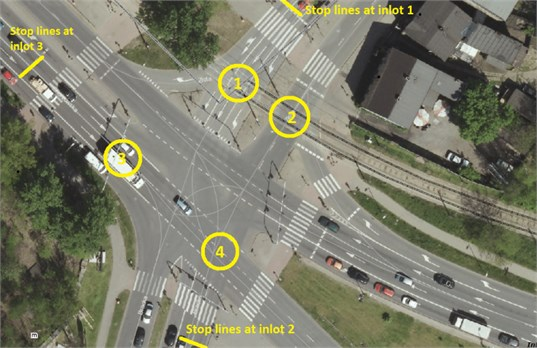In Fig. 3, four measuring points, in which car vehicle speed measurements were performed, have been marked in the circles. Therefore:

• in measurement point No. 1, the speeds of the car vehicles entering from inlet No. 1, going straight and passing through the tram tracks have been recorded (measurement at a distance of 10 m from the stop line),

• in measurement point No. 2, the speeds of the car vehicles entering from inlet No. 2, going straight and passing through the tram tracks have been recorded (measurement at a distance of 30 m from the stop line),

• in measurement point No. 3, the speeds of the car vehicles entering from inlet No. 3, going straight and not passing through the tram tracks have been recorded (measurement at a distance of 10 m from the stop line),

• in measurement point No. 4, the speeds of the car vehicles entering from inlet No. 3, going straight and not passing through the tram tracks have been recorded (measurement at a distance of 30 m from the stop line).

The study was conducted on one of the days of the week, representative for the carrying out traffic measurements [35-37]. The measurements have been carried out using a video camera and a Bushnell Velocity manual device (series 101911). The measurement with the use of a video camera was used to determine traffic volumes, as well as to identify queues of car vehicles and the generic structure of the traffic. In turn, the Bushnell Velocity device was used to measure the instantaneous vehicle speed. The measurement accuracy of this device is +/–2 km/h. The speed has been recorded for the first six vehicles in the queue. Position the vehicle was determined at the begin of the green signal.

## 4. Result of research and their analysis

To determine the speed distributions in relation to the vehicle positions in the queue for individual measurement points, only the signaling cycles were analyzed, in which a homogeneous generic structure of traffic has been observed (only passenger cars were present).

### 4.1. Distribution of instantaneous vehicle speeds – results for each measurement point

Fig. 4 present the distribution of instantaneous vehicle speeds for individual measurement points in relation to the vehicle position in the queue.

### 4.2. Distribution of instantaneous vehicle speeds – results for each measurement point

The test results presented in Tables 1 to 4 show the average speed for successive vehicles in the queue, standard deviation and coefficient of variation as well as the size of the sample, which was the basis for determining these statistical measures. In turn, in Figs. 5 to 8 the relationships between the average speed and the position of the vehicle in the queue for individual measurement points have been shown.

Table 1Analysis of average speed for successive vehicles in the queue for measurement point No. 1 (measurement at a distance of 10 m from the stop line for car vehicle passing through the tram tracks)

 Vehicle position in the queue Size of the sample [–] Average speed [km/h] Standard deviation of the speed [km/h] Coefficient of variation [–] 1 60 19,1 2,6 0,136 2 60 20,1 2,8 0,139 3 60 21,2 3,6 0,170 4 54 21,1 3,1 0,147 5 50 21,4 2,8 0,131 6 42 21,9 2,8 0,128

Table 5 presents mathematical models that describe the dependence of average speed on the position of car vehicle in the queue for the analyzed cases.

For each measurement point, four types of mathematical models were formulated, i.e.: exponential, linear, logarithmic, and power. The coefficient of determination was assumed as a measure of the choice of the best-fit model. For three measurement points (except measurement point No. 4), the values of the coefficient indicate strong correlation. Functions with the largest values of the correlation coefficient are: logarithmic for measurement point No. 1 (${R}^{2}=$ 0,9578) and for measurement point No. 2 (${R}^{2}=$ 0,9986). In turn, for measurement point No. 3 (${R}^{2}=$ 0,969) it is a linear function.

Fig. 4Distribution of instantaneous vehicle speeds for individual measurement points in relation to the vehicle position in the queue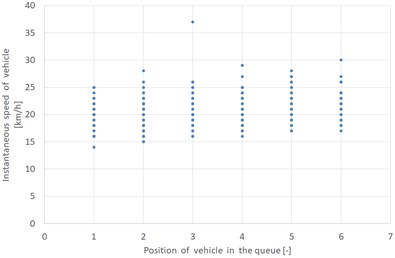a) Measurement point No. 1 – passing through the tram tracks (measurement at a distance of 10 m from the stop line)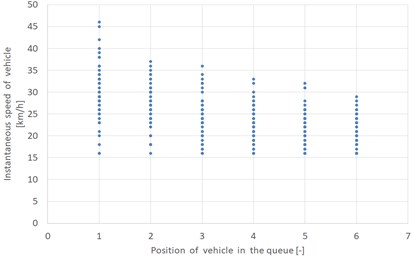b) Measurement point No. 2 – passing through the tram tracks (measurement at a distance of 30 m from the stop line)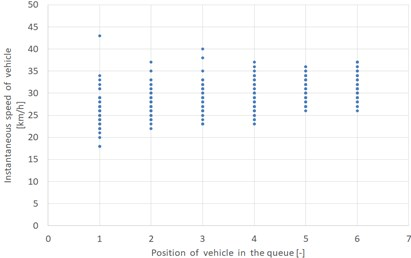c) Measurement point No. 3 – without passing through the tram tracks (measurement at a distance of 10 m from the stop line)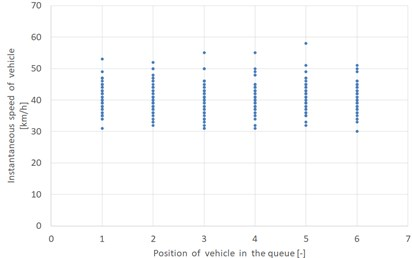d) Measurement point No. 4 – without passing through the tram tracks (measurement at a distance of 30 m from the stop line)

Fig. 5Results for measurement point No. 1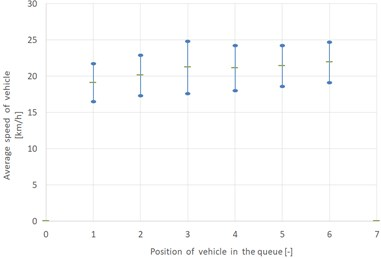a) Average speed in relation to the position of the car vehicle in the queue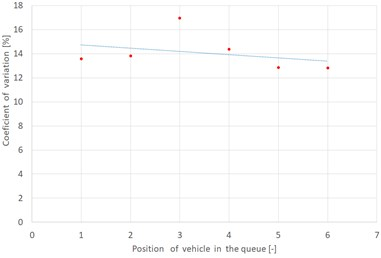b) Coefficient of variation in relation to the position of the car vehicle in the queue

However, for measurement point No. 4, the value of the coefficient of determination indicates the lack of correlation between the average vehicle speed and the position of the vehicle in the queue. For each of the analyzed mathematical models the value of the coefficient of determination is small, not exceeding 0,1. However, this is the natural phenomenon, because the measurement took place at a distance of 30 m from the stop line for vehicles not passing through the tram track, then the drivers reach their intended speed.

Table 2Analysis of average speed for successive vehicles in the queue for measurement point No. 2 (measurement at a distance of 30 m from the stop line for car vehicle passing through the tram tracks)

 Vehicle position in the queue Size of the sample [–] Average speed [km/h] Standard deviation of the speed [km/h] Coefficient of variation [–] 1 59 29,7 6,2 0,209 2 59 26,5 4,8 0,181 3 59 24,3 4,7 0,193 4 59 22,8 4,0 0,175 5 59 21,8 4,0 0,183 6 59 21,1 3,5 0,166

Fig. 6Results for measurement point No. 2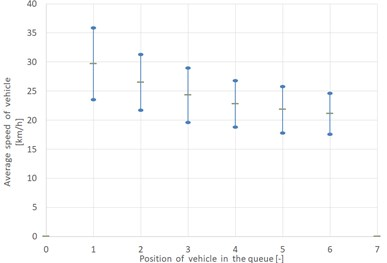a) Average speed in relation to the position of the car vehicle in the queue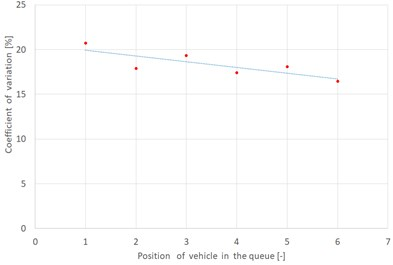b) Coefficient of variation in relation to the position of the car vehicle in the queue

Table 3Analysis of average speed for successive vehicles in the queue for measurement point No. 3 (measurement at a distance of 10 m from the stop line for car vehicle not passing through the tram tracks)

 Vehicle position in the queue Size of the sample [–] Average speed [km/h] Standard deviation of the speed [km/h] Coefficient of variation [–] 1 59 26,2 3,9 0,149 2 59 27,9 3,0 0,108 3 59 28,6 3,6 0,126 4 59 29,0 3,4 0,117 5 59 30,7 2,7 0,088 6 59 31,4 2,8 0,089

Fig. 7Results for measurement point No. 3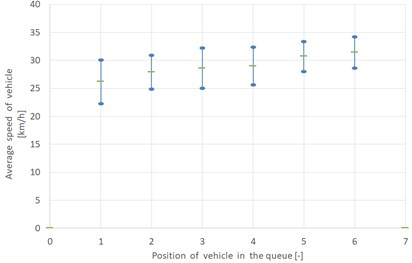a) Average speed in relation to the position of the car vehicle in the queue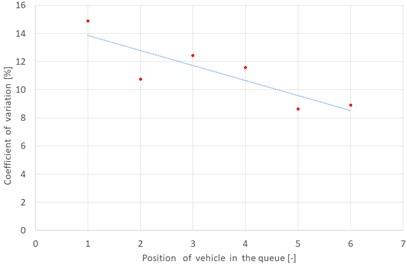b) Coefficient of variation in relation to the position of the car vehicle in the queue

Table 4Analysis of average speed for successive vehicles in the queue for measurement point No. 4 (measurement at a distance of 30 m from the stop line for car vehicle not passing through the tram tracks)

 Vehicle position in the queue Size of the sample [–] Average speed [km/h] Standard deviation of the speed [km/h] Coefficient of variation [–] 1 61 40,5 4,4 0,109 2 61 40,5 4,3 0,106 3 61 40,0 4,7 0,118 4 61 40,7 4,3 0,106 5 61 41,0 4,5 0,110 6 61 40,4 4,1 0,101

Fig. 8Results for measurement point No. 4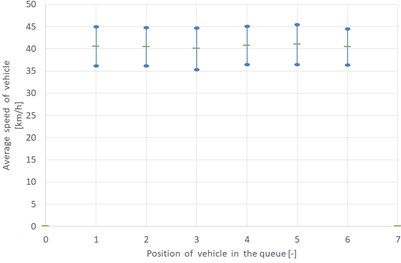a) Average speed in relation to the position of the car vehicle in the queue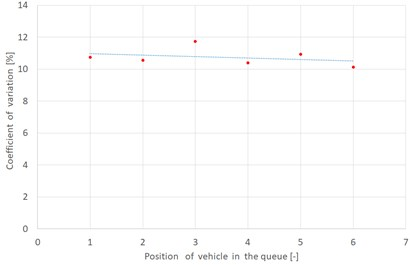b) Coefficient of variation in relation to the position of the car vehicle in the queue

Table 5Mathematical models describing the dependence of average speed on the position of car vehicle in the queue

 No Name of the analyzed case (measurement point) Type of function Mathematical model Coefficient of determination ${R}^{2}$ 1 Measurement at a distance of 10 m from the stop line for car vehicle passing through the tram tracks(measurement point No. 1) Exponential ${V}_{śr}^{+t}=\text{19,053}{e}^{0,0248\bullet n}$ 0,8618 Linear ${V}_{śr}^{+t}=\text{0,5086}n+$ 19,02 0,8704 Logarithmic ${V}_{śr}^{+t}=\text{1,5062}\mathrm{l}\mathrm{n}\left(n\right)+$ 19,148 0,9578 Power ${V}_{śr}^{+t}=\text{19,165}{n}^{0,0737}$ 0,9576 2 Measurement at a distance of 30 m from the stop line for car vehicle passing through the tram tracks(measurement point No. 2) Exponential ${V}_{śr}^{+t}=\text{30,631}{e}^{-0,067\bullet n}$ 0,9525 Linear ${V}_{śr}^{+t}=-\text{1,674}n+$ 30,227 0,9306 Logarithmic ${V}_{śr}^{+t}=-\text{4,897}\mathrm{l}\mathrm{n}\left(n\right)+$ 29,736 0,9986 Power ${V}_{śr}^{+t}=\text{29,95}{n}^{-0,195}$ 0,9966 3 measurement at a distance of 10 m from the stop line for car vehicle not passing through the tram tracks(measurement point No. 3) Exponential ${V}_{śr}^{-t}=\text{25,63}{e}^{0,0345\bullet n}$ 0,9659 Linear ${V}_{śr}^{-t}=\text{0,9943}n+$ 25,487 0,9690 Logarithmic ${V}_{śr}^{-t}=\text{2,757}\mathrm{l}\mathrm{n}\left(n\right)+$ 25,943 0,9349 Power ${V}_{śr}^{-t}=\text{26,018}{n}^{0,0963}$ 0,9462 4 measurement at a distance of 30 m from the stop line for car vehicle not passing through the tram tracks(measurement point No. 4) Exponential ${V}_{śr}^{-t}=\text{40,339}{e}^{0,0012\bullet n}$ 0,0779 Linear ${V}_{śr}^{-t}=\text{0,05}n+$ 40,339 0,0786 Logarithmic ${V}_{śr}^{-t}=\text{0,1072}\mathrm{l}\mathrm{n}\left(n\right)+$ 40,396 0,0453 Power ${V}_{śr}^{-t}=\text{40,396}{n}^{0,0026}$ 0,0446 ${V}_{śr}^{+t}$ – average speed of car vehicles, passing through the tram tracks; ${V}_{śr}^{-t}$ – average speed of car vehicles, not passing through the tram tracks; $n$ – position of car vehicle in the queue ($n\in N$).

The best-fit models for all measurement points have been shown in Fig. 9.

Fig. 9The best-fit models for all measurement points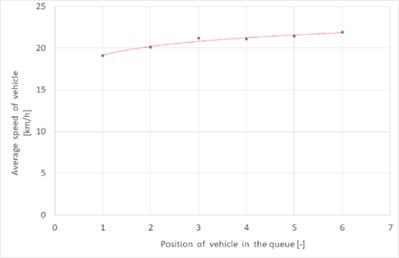a) Measurement point No. 1 – passing through the tram tracks (measurement at a distance of 10 m from the stop line)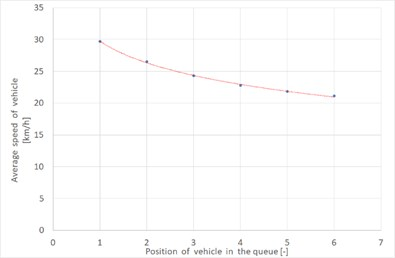b) Measurement point No. 2 – passing through the tram tracks (measurement at a distance of 30 m from the stop line)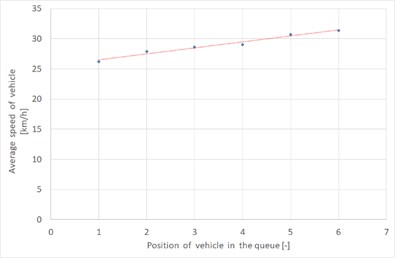c) Measurement point No. 3 – without passing through the tram tracks (measurement at a distance of 10 m from the stop line)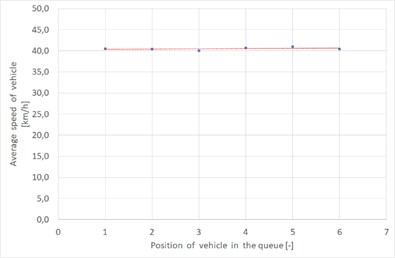d) Measurement point No. 4 – without passing through the tram tracks (measurement at a distance of 30 m from the stop line)

### 4.3. Comparison of the average speed of vehicles passing through the tram tracks with the average speed of vehicles not passing through the tram tracks

The main aim of research performed at different distances from tram tracks was to compare and to analyze the impact of tram tracks on speed in relations to the position of the vehicle in the queue. Fig. 10 presents the distribution of average speeds in relation to the individual positions in the queue for vehicle, which passed through the tram tracks and for those that did not pass the tracks at a distance of 10 meters from the stop line.

Fig. 10 shows the impact of tram tracks located at a distance of 10 m from the stop line on the speed of car vehicles. The values of the tested feature for both vehicles passing through and vehicles non-passing through the tram tracks increase for successive vehicles in the queue. In addition, the differences between the speeds of vehicles non-passing through the tram tracks and non-passing tracks increase for each subsequent vehicle in the queue. In addition, the differences between the speeds of vehicles not passing through the tram tracks and the speeds of vehicles passing the tracks are growing up for each subsequent vehicle in the queue. For the first vehicle in the queue, the value of this difference 7.1 [km/h] and for the sixth vehicle – 9.5 [km/h]. This tendency may be seen in Fig. 12.

Fig. 11 presents the distribution of average speeds in relation to the individual positions in the queue for vehicle, which passed through the tram tracks and for those that did not pass the tracks at a distance of 30 meters from the stop line.

Fig. 11 shows the impact of the occurrence of the tram tracks on the speeds of vehicles located within 30 m from the stop line. The values of the average speeds of vehicles passing through the tram tracks indicate the need to slow down before them. In turn, in the case of absence of the tram tracks, there are no observed declines in the speed associated with the need for braking before the tracks, because the drivers of vehicles reach their intended speed.

Fig. 10The best-fit models for all measurement points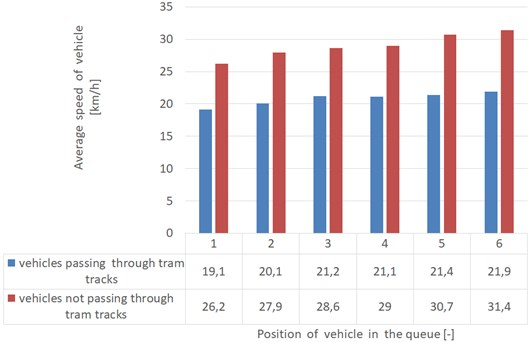Fig. 11The comparison of average speed for each position of vehicles in the queue – 30 m from stop line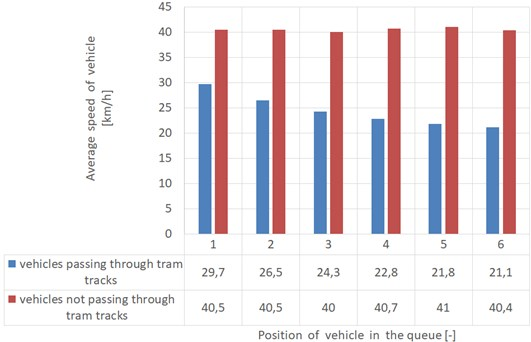Therefore, it is observed that the differences $∆{V}_{sr}$ between the average speeds of vehicles passing through the tram tracks $\left({V}_{sr}^{+t}\right)$ and speeds of vehicles non-passing through the tracks $\left({V}_{sr}^{-t}\right)$ increase for each subsequent vehicle in the queue. For the first vehicle in the queue the value of the discussed difference is 10.8 [km/h] and for the sixth vehicle – 19.3 [km/h] (for the distance of 30 m from the stop line). Fig. 12 shows the differences $∆{V}_{sr}$ in relation to the position of the vehicle in the queue for two measurement distances from the stop line. Based on the measurement results, it is possible to build mathematical models presented in Table 6.

Table 6Mathematical models describing the dependence of differences between average speed of vehicles passing through the tram tracks and speed of vehicles not passing the tracks on the position of car vehicle in the queue

 No. Distance from the stop line [m] Mathematical model Coefficient of determination ${R}^{2}$ 1 10 ${\mathrm{\Delta }V}_{śr}=6,6132{e}^{0,0585\bullet n}$ 0,8324 2 30 ${\mathrm{\Delta }V}_{śr}=5,0076\mathrm{l}\mathrm{n}\left(n\right)+10,659$ 0,9878 , ${V}_{śr}^{-t}$ – average speed of car vehicles, non-passing through the tram tracks; ${V}_{śr}^{+t}$ – average speed of car vehicles, passing through the tram tracks; $n$ – position of car vehicle in the queue ($n\in N$).

It may be seen that these differences are greater when the measurement took place at a distance of 30 m than at a distance of 10 m.

Fig. 12Differences between average speed of vehicles passing through the tram tracks and speed of vehicles not passing the tracks for two distances from stop line: 10 m and 30 m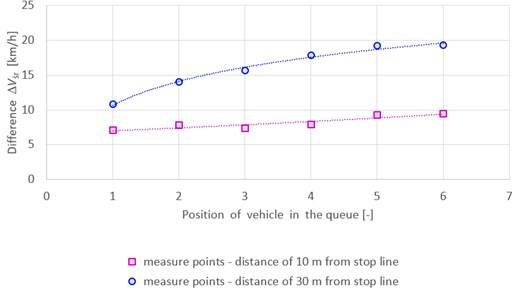## 5. Noise emission in terms of charge of the speed of car vehicles passing through tram tracks

Considerations regarding noise emissions are particularly important due to the quality of life of urban residents and its direct impact on their health and well-being. In addition, the results of research related to noise emission are especially relevant from the point of view of designing new and modernizing existing transportation systems. The use of predictive models to determine the level of noise emission from a given transportation system allows for shaping it in accordance with the idea of sustainable development. This applies to both systems in urban and non-urban areas [38-40].

The speed change caused by passing through the tram tracks induces a change in the noise emission level. Based on the conducted research, it is possible to determine the impact of such situation on the noise emitted under different conditions.

When analyzing the available models of noise emission from road traffic, it may be concluded that in most cases they accurately reflect the actual noise levels [41, 42]. By adopting one of the methods for estimating noise emission, it is possible to determine the hourly level of its intensity for selected assumptions. The considerations in this issue have been based on the FHWA model . This model allows to determine the noise level as an hourly average sound pressure emitted by a passing vehicle of $i$th type.

According to the methodology included in the description of the FHWA model, noise emission estimation is based on the following relationship (Eq. (3)):

3
${L}_{eq\left(h\right)i}={L}_{0}+0,115{s}^{2}+10\mathrm{l}\mathrm{o}\mathrm{g}\left(\frac{{N}_{i}p{D}_{o}}{{S}_{i}T}\right)+10\mathrm{l}\mathrm{o}\mathrm{g}{\left(\frac{{D}_{o}}{D}\right)}^{1+\alpha }$

where ${L}_{0}$ – basic noise value determined for a distance of 15 m from the road above ground level [dB], ${N}_{i}$ – volume of traffic, consisting of vehicles of $i$th type [veh/h], ${S}_{i}$ – average speed of vehicles of $i$th class [km/h], $D$ – distance from the calculation point to the traffic lane in the orthogonal projection [m], ${D}_{0}$ – distance from the center of the road to the point of calculation [m], $T$ – the period of time, for which the noise level is calculated [h], $\alpha$ – parameter of side, it ranges between 0 and 1, ${\phi }_{1}$, ${\phi }_{2}$ – angles between the line perpendicular to the road section from the calculation point, they take values from the range <–90; 90>, $\mathrm{\Delta }s$ – correction factor due to barriers such as buildings or screens.

The total noise level for all vehicle types may be determined by the formula (Eq. (4)):

4

where ${L}_{eqC}$ – noise level of passenger car vehicles, – the noise level of light goods vehicles, ${L}_{eqHGV}$ – the noise level of heavy goods vehicles.

Therefore, in order to conduct an analysis of the impact of speed change on the noise change for different cases, general assumptions have been made, as shown in Table 7. The parameter of side was set at 0.5 and the angles at –90 and 90 degrees. Table 8 presents the results obtained from the model for defined variants. In turn, the graph in Fig. 13 depicts differences in noise levels. The calculations have been carried out for four speed levels, i.e. for the first vehicles in the queue at a distance of 10 m and 30 m from the stop line, separately for the car vehicles passing through the tram tracks and non-passing through the tram tracks.

Table 7Variants and assumed values of selected parameters necessary to determine the noise level

 W1 W2 W3 W4 Passenger car vehicles – C [veh/h] 400 400 400 400 Light goods vehicles – LGV [veh/h] 20 20 20 20 Heavy goods vehicles – HGV [veh/h] 10 10 10 10 Speed [km/h] 19,1 26,7 29,7 40,5 Distance $D$ [m] 15 15 15 15 Distance ${D}^{\text{'}}$ [m] 25 25 25 25 Distance ${D}^{\text{'}\text{'}}$ [m] 40 40 40 40

Table 8Noise emission levels for defined variants

 Estimated parameters Distance W1 W2 W3 W4 ${L}_{eqT}$[dB] $D$ 58,0 60,9 61,8 64,7 ${D}^{\text{'}}$ 54,7 57,5 58,5 61,3 ${D}^{\text{'}\text{'}}$ 51,6 54,5 55,4 58,3 ${L}_{eqC}$[dB] $D$ 51,1 55,2 56,5 60,03 ${D}^{\text{'}}$ 47,8 51,8 53,1 56,9 ${D}^{\text{'}\text{'}}$ 44,7 48,8 50,1 53,9 ${L}_{eqLGV}$[dB] $D$ 50,6 54,1 55,2 58,4 ${D}^{\text{'}}$ 47,3 50,8 51,9 55,1 ${D}^{\text{'}\text{'}}$ 44,2 47,7 48,8 52,0 ${L}_{eqHGV}$[dB] $D$ 55,9 58 58,7 60,6 ${D}^{\text{'}}$ 52,6 54,7 55,4 57,3 ${D}^{\text{'}\text{'}}$ 49,5 51,6 52,3 54,3

Fig. 13Noise emission levels for determined variants according to individual vehicle types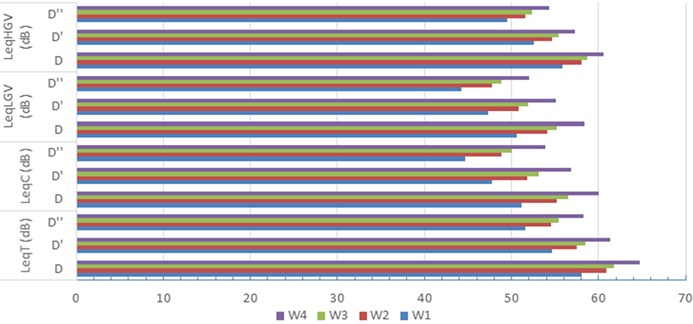Reduction of speed resulting from the necessity of the passing by the car vehicle through the tram tracks also reduces the emitted noise. However, this is a relatively small change. It should be emphasized that these conclusions have been formulated only on the basis of speed change. Further detailed studies are needed to correct the determined noise emission levels. It should be taken into account that the passing through the tram tracks also generates additional noise. Research in this direction would allow more objectively estimate the total noise emitted both by the passing through the tram tracks and by the reduction in speed. It is possible that the decreasing of noise due to the speed reduction will be compensated or even exceeded by the noise generated as a result of passing through the tracks. However, confirmation of the above statements requires additional empirical studies conducted in real traffic conditions.

## 6. Conclusions

The subject of the analysis was the impact of tram tracks situated at a distance of 10 and 30 meters from the stop line on the speed of vehicles passing through the intersection located on multilane artery in the city. Based on the conducted research, it was found that the presence of tram tracks causes a decrease in the speed of car vehicles, which is dependent on the position of the vehicle in the queue at the beginning of the green signal and on the distance of the stop line from the tracks.

The analysis of mathematical models presented in Table 5 allows to state that for measurements carried out at a distance of 10 m from the stop line (regardless of passage through the tracks), the speed increases exponentially for each vehicle position in the queue. Subsequent vehicles waiting in the queue for the green signal travel more and more distance to the measurement point, so they may develop a higher speed. However, the presence of a tram tracks results in a decrease in the average speed of vehicles in the analyzed cross-section. For example, according to a model that takes into account the passage of vehicles through a tram tracks, the first vehicle crosses the measurement point at 18,65 km/h. In turn, according to the model, which does not take into account the passage through the tracks, the first vehicle in the queue exceeds the cross-section with the speed of 25,22 km/h.

The results of measurements carried out at a distance of 30 m from the stop line show different relationships between the variables studied. There is a decrease in speed with the increase of the position of the vehicle in the queue. Drivers passing through the tram tracks reduce their speed directly in front of them, trying to keep a safe distance to the vehicle ahead. Such knowledge may be used, among others, while planning temporary traffic organization [43, 44] and conducting road safety analyzes [45, 46].

For comparison of the speed distribution at different measurement points, the arithmetic mean and the standard deviation were determined. Small values of the coefficient of variation allow to take the average values as reliable. Therefore, it may be stated that the measurements reflect the actual traffic conditions occurring on the tested intersection.

The presented research results confirmed the impact of tram tracks on the speed of vehicles. However, to be able to generalize this thesis, similar tests should be carried out at other intersections with traffic lights and tram tracks. In addition, it is worth investigating the relationships between vehicle speed and other factors associated with tram tracks (e.g. distance between tracks and stop line, quality of tracks). Therefore, the issue presented in the article opens up a wide field of research. The test results should be included in the update of the method of estimating intersection capacity with traffic lights used in Poland.

#### Cited by

The use of the risk matrix method for assessing the risk of implementing rail freight services
Traffic Light Priority for Trams in Warsaw as a Tool for Transport Policy and Reduction of Energy Consumption
Effective Method for Diagnosing Continuous Welded Track Condition Based on Experimental Research
Monitoring System for Railway Infrastructure Elements Based on Thermal Imaging Analysis
Risk assessment for rail freight transport operations
Simulation of environmental pollution from diesel locomotive